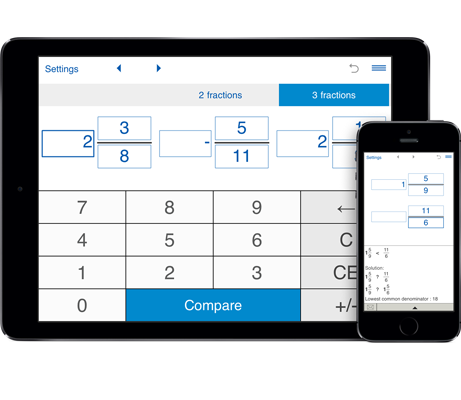# Compare fractions

Compares proper and improper fractions and mixed numbers.
You can use this calculator to compare two and three fractions.
In case of comparing 3 fractions, the calculator arranges them in order from least to greatest.
Compares fractions with like and unlike denominators.

## Compare fractions calculator

### Comparing fractions and mixed numbers

This compare fractions calculator will compare two or three fractions to find out if they are equal, greater or less than each other. With this calculator you can compare fractions with the same and different denominators.

#### Comparing fractions with like denominators:

To compare fractions with like denominator the caluclator compares their numerators to see which fraction is greater.
When comparing two fractions with like denominators, the fraction with larger numerator is greater. Note: mixed numbers are converted to improper fractions first.

#### Comparing fractions with unlike denominators:

To compare fractions with different denominators, the calculator finds the least common denominator (LCD) of fractions, converts the fractions to equivelant fractions with a common denominator, then compares the numerators. The larger fraction is the one with the greater numerator. To find the least common denominator (LCD) of fractions, the caluclator finds the least common multiple (LCM) of their denominators.

#### How to compare 2 or 3 fractions

1. Select "2 fractions" or "3 fractions".
2. Input the fractions. Enter the whole number, numerator and the denominator (interger values).
3. Click on "Compare".
In case of comparing 3 fractions, the calculator arranges them in order from least to greatest.